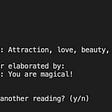# The Prompt

Create a function that takes in a Binary Search Tree (BST), it may be invalid, and returns a boolean value representing whether the BST is valid.

Each BST node has an integer value, a left child node, and a right child node.

A node is valid if it satisfies the BST property: its value is greater than every node to it’s left, and its value is less than every node to its right.

# The Code

Let’s start off by initiating the BST class and creating a template for our solution.

Now, the validateBst function takes in only a tree as its input, but the logic behind the validation requires a minimum and maximum value, to act as pointers. Therefore, we’ll create a helper function that will take in the parameters we need in order to get our final solution. Let’s start.

The beginning logic can go as the following:

• if there is no Tree, return TRUE
• if the tree’s value is less than the minimum value (minVal) OR is greater than the maximum value (maxVal), then return FALSE

Below is an example of how the code in the helper function should look like so far.

Now, we can create a variable that will act as checker for the left value, then apply the same logic to check the right value. This can be done using recursion.

Now that the helper function is complete, simply invoke the helper function in our initial validateBst function, and the solution is complete. The final code should look like the following:

# Complexity Analysis

The time complexity of this solution will be O(n) time, where (n) is the length of the BST.

The space complexity of this solution will be O(d) space, where (d) is the depth of the BST, since we’re using recursion and applying the helper function to each of the child nodes.

--

--

--

## More from Karan S. Chauhan

Love podcasts or audiobooks? Learn on the go with our new app.

## Wait, what is my fleet doing?## Getting started in Apron Network Bug Bounty Program## The Rendering Equation Explained## Box Open With UI Element available now in beta, featuring G Suite and Adobe Sign## WHAT IS MACHINE LEARNING ?